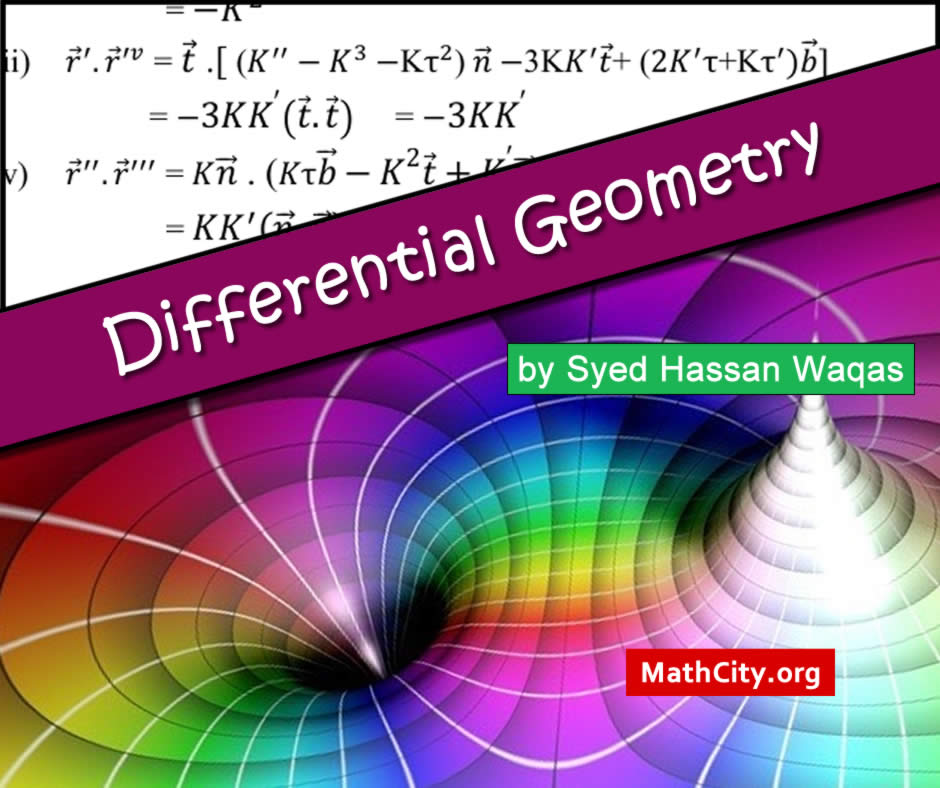# Differential Geometry by Syed Hassan WaqasIn the field of mathematics known as differential geometry, smooth manifolds—also known as smooth shapes and spaces—and their geometry are studied. Investigating the geometric characteristics of curves and surfaces involves the use of the tools of differential and integral calculus, linear algebra, and multilinear algebra. Differential calculus and mathematical analysis are related to differential geometry.

These notes are provided and composed by Mr. Muzammil Tanveer. We are really very thankful to him for providing these notes and appreciates his effort to publish these notes on MathCity.org

 Name Differential Geometry Mr. Muzammil Tanveer 69 pages PDF (see Software section for PDF Reader) 2.7 MB
• Curvature
• Formula for Radius of Curvature
• Centre of Curvature
• Curvature and Radius of Curvature in Polar Form
• Space curve or Twisted curve or Skew curve
• Tangent line
• Normal line
• Secant line
• Equation of tangent of a point
• Normal plane
• Equation of Normal plane
• Oscillating plane or plane of Curvature
• Frenet-Serret Formula
• Torsion
• arameters other than ‘s’
• Equation of the Centre of Curvature
• Moving Trihedral
• Helix
• Spherical indicatrices
• Spherical Indicatrices of tangent
• Spherical Indicatrices of Normal
• Spherical Indicatrices of Binormal
• Surface
• Another definition of Surface
• Tangent plane
• Equation of Normal
• Parametric curves
• U-curves
• V-curves
• First order Fundamental magnitude
• Second order Fundamental form and second order Fundamental magnitude

• notes/differential-geometry-syed-hassan-waqas